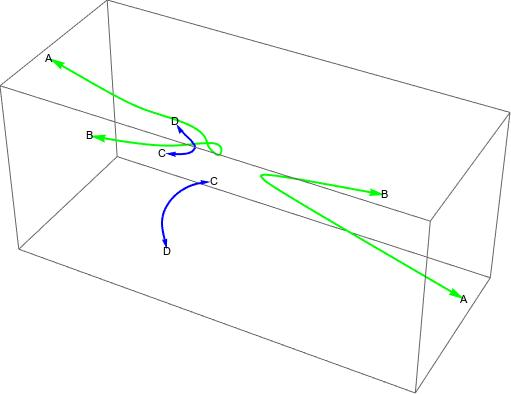# Space Curve Book

## Barry H DaytonA curve in 4-space

Space curves in n-dimensional affine or projective space present a major challenge that we did not need to deal with for plane curves, instead of a single equation a system of n-1 or more equations is needed. This system is far from unique and, in many cases, may be over-determined. Since I allow numerical coefficients and, in general, numerical over-determined systems are to be avoided, special methods must be used.

The book is available here: SpaceCurveBook_v2c (PDF 3.1MB) . Please note that this PDF is optimized for screen viewing. It will not print efficiently on paper. While minor corrections or additions may be posted this is basically the complete final version.

I first discuss naive space curves in 3-space, that is, curves defined by a system of two equations in 3 variables. Not only is this case familiar to what we learned in multivariable calculus but many methods in the plane curve case such as critical points and path tracing are still available.

Then I develop the methods from numerical linear algebra that will be needed: Macaulay and Sylvester matrices and duality. The basic definitions and techniques for the general case will then be established.

The last part will cover, as examples, various situations I have recently presented in papers and talks. It should be noted that material from Section 3.1 is now available separately in the Mathematica Journal vol 22 Degree versus Dimension for Rational Parametric Curves

In addition here is a Mathematica 12.1 notebook with code and examples for the global functions MD used in this book along with an alphabetical list of the fuctions (PDF) with their syntax.

Please note there was a bug in `nDivideMD` which has now been fixed. To use functions `nDivideMD, nGCDMD, SqFreeMD` make sure you use a version of GlobalFunctionsMD.nb downloaded after January 2021.

1. Chapter 1: Naive Curves: Curves in 3-Space with 2 equations
• 1.1 Emulating Plane Curves
• 1.2 Projections
• 1.3 Ovals and Pseudo Lines
• 1.4 Fractional Linear Transformations on 3-Space
2. Chapter 2: General Case -- Theory
• 2.1 The twisted Cubic
• 2.2 Tangent Vectors and definition of Curve
• 2.3 Macaulay and Sylvester Matrices
• 2.4 H-Bases
• 2.5 Duality, Intersections, Unions and Decomposition
• 2.6 Fractional Linear Transformations
• 2.7 Geometry and Projections
• 2.8 Fibers and plotting space curves
• 2.9 Fundamental Theorem
• 2.10 Bezout's Theorem
3. Chapter 3: Applications
• 3.1 Implicitization
This note is an exposition of Theorem 1.18 in Joe Harris' Algebraic Geometry book which says that given d + 3 points in general position in projective d-space there is a unique degree d rational normal curve passing through these points. A rational normal curve (RNC) is a curve projectively equivalent to the projective closure of the parametric rational normal curve `{td,t,d-1,...,t}` Harris' exposition is a bit sparse on implementation details and does not adequately address the uniqueness. I will be able to explain uniqueness heuristically, although will not give a formal proof except for d = 2. In addition I will be able to explain why the hypotheses on general position is necessary and sufficient for a set of d + 3 points to be on a RNC. I will also give insight as to why d+3 is the correct number. This is presumably a classical result but other expositions I have seen seem to follow Harris. As always I will use a numerical approach with Mathematica.